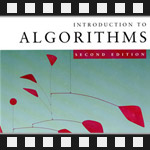This is the seventh post in an article series about MIT's lecture course "Introduction to Algorithms." In this post I will review lecture eleven, which is on the topic of Augmenting Data Structures.

There are some programming situations that can be perfectly solved with standard data structures such as a linked lists, hash tables, or binary search trees. Many others require a dash of creativity. Only in rare situations will you need to create an entirely new type of data structure, though. More often, it will suffice to augment (to modify) an existing data structure by storing additional information in it. You can then program new operations for the data structure to support the desired application. Augmenting a data structure is not always straightforward, however, since the added information must be updated and maintained by the ordinary operations on the data structure.

This lecture discusses two data structures that are constructed by augmenting red-black trees (see the previous post on red-black trees).

The first part of the lecture describes a data structure that supports general order-statistic operations on a dynamic set. It's called dynamic order statistics. The notion of order statistics was introduced in lecture six. In lecture six it was shown that any order statistic could be retrieved in O(n) time from an unordered set. In this lecture it is shown how red-black trees can be modified so that any order statistic can be determined in O(lg(n)) time. It presents two algorithms OS-Select(i), which returns i-th smallest item in a dynamic set, and OS-Rank(x), which returns rank (position) of element x in sorted order.

The lecture continues with general methodology of how to augment a data structure. Augmenting a data structure can be broken into four steps:

• 1. Choosing an underlying data structure,
• 2. Determining additional information to be maintained in the underlying data structure,
• 3. Verifying that the additional information can be maintained for the basic modifying operations (insert, delete, rotate, etc.) on the underlying data structure, and
• 4. Developing new operations.

The second part of the lecture applies this methodology to construct a data structure called interval trees. This data structure maintains a dynamic set of elements, with each element x containing an interval. Interval is simply pair of numbers (low, high). For example, a time interval from 3 o'clock to 7 o'clock is a pair (3, 7).

Lecture gives an algorithm called Interval-Search(x), which given a query interval x, quickly finds an interval in the set that overlaps it. Time complexity of this algorithm is O(lg(n)).

The lecture ends with the correctness proof of Interval-Search(x) algorithm.

You're welcome to watch lecture eleven:

Topics covered in lecture eleven:

• [00:20] Concept of augmenting data structures.
• [02:00] Dynamic order statistics.
• [02:20] OS-Select operation on dynamic order statistics.
• [02:50] OS-Rank operation on dynamic order statistics.
• [03:49] Dynamic order statistics key idea - keep the sizes of subtrees in nodes of a red-black tree.
• [04:10] Example of a tree representing dynamic order statistic.
• [10:10] OS-Select algorithm.
• [16:40] Analysis of OS-Select.
• [17:30] OS-Rank algorithm.
• [20:15] Modifying operations of dynamic order statistics tree.
• [22:55] Example of inserting an element into the tree.
• [26:11] Example of rotating a tree.
• [29:30] Methodology of data structure augmentation.
• [36:45] Data structure augmentation applied to construct interval trees.
• [37:31] Example of time-intervals.
• [39:48] Query operation on interval trees - find an interval in the set that overlaps a given query interval.
• [41:15] Step 1, underlying data structure: red-black tree keyed on low endpoint.
• [45:10] Step 2, additional node information: largest value in the subtree rooted at that node.
• [50:24] Step 3, modifying ops: insert, delete.
• [56:55] Step 4, new ops: Interval-Search.
• [01:00:00] Example of Interval-Search algorithm.
• [01:06:30] Running time of Interval-Search -- O(lg(n)).
• [01:07:20] List all overlaps (k of them) in O(k*lg(n)) time.
• [01:08:50] Best algorithm to find all overlaps to date os O(k + lg(n)).
• [01:09:11] Correctness proof of Interval-Search algorithm.

Lecture eleven notes:

Have fun augmenting data structures! The next post will be about a simple and efficient search structure called skip list.